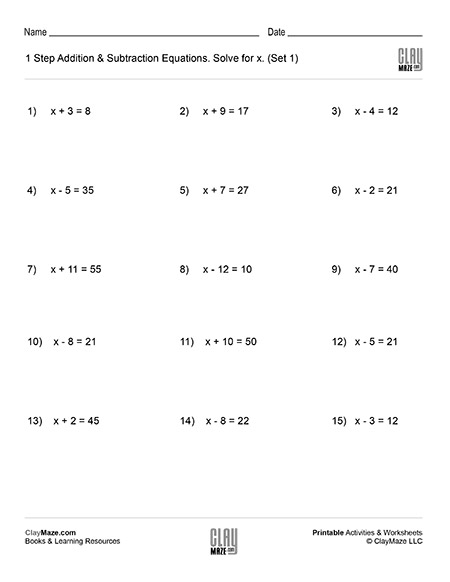# Algebra: 1 Step Addition & Subtraction Equations (Set 1)

This worksheet contains problems that act as an introduction to algebra. The problems are 1 step addition and subtraction equations that ask the student to solve for x. – solutions included